# Mean State

Period Mean (original grids) [W/m2]
Model Period Mean (intersection) [W/m2]
Model Period Mean (complement) [W/m2]
Benchmark Period Mean (intersection) [W/m2]
Benchmark Period Mean (complement) [W/m2]
Bias [W/m2]
RMSE [W/m2]
Phase Shift [months]
Bias Score 
RMSE Score 
Seasonal Cycle Score 
Spatial Distribution Score 
Interannual Variability Score 
Overall Score 
Benchmark [-] 218.
CLM5PHSOFF [-] 216. 215. 0.00 218. 219. -2.67 17.1 0.845 0.74 0.49 0.90 0.94 0.82 0.73
CLM5PHSON [-] 215. 215. 0.00 218. 219. -2.67 17.1 0.845 0.74 0.49 0.90 0.94 0.82 0.73
Period Mean (original grids) [W/m2]
Model Period Mean (intersection) [W/m2]
Model Period Mean (complement) [W/m2]
Benchmark Period Mean (intersection) [W/m2]
Benchmark Period Mean (complement) [W/m2]
Bias [W/m2]
RMSE [W/m2]
Phase Shift [months]
Bias Score 
RMSE Score 
Seasonal Cycle Score 
Spatial Distribution Score 
Interannual Variability Score 
Overall Score 
Benchmark [-] 243.
CLM5PHSOFF [-] 240. 241. 0.00 243. 228. -2.41 14.7 0.393 0.81 0.55 0.97 0.99 0.86 0.79
CLM5PHSON [-] 240. 241. 0.00 243. 228. -2.41 14.7 0.393 0.81 0.55 0.97 0.99 0.86 0.79
Period Mean (original grids) [W/m2]
Model Period Mean (intersection) [W/m2]
Model Period Mean (complement) [W/m2]
Benchmark Period Mean (intersection) [W/m2]
Benchmark Period Mean (complement) [W/m2]
Bias [W/m2]
RMSE [W/m2]
Phase Shift [months]
Bias Score 
RMSE Score 
Seasonal Cycle Score 
Spatial Distribution Score 
Interannual Variability Score 
Overall Score 
Benchmark [-] 233.
CLM5PHSOFF [-] 232. 232. 0.00 233. 234. -0.710 16.8 0.786 0.84 0.57 0.90 0.98 0.76 0.77
CLM5PHSON [-] 232. 232. 0.00 233. 234. -0.710 16.8 0.786 0.84 0.57 0.90 0.98 0.76 0.77
Period Mean (original grids) [W/m2]
Model Period Mean (intersection) [W/m2]
Model Period Mean (complement) [W/m2]
Benchmark Period Mean (intersection) [W/m2]
Benchmark Period Mean (complement) [W/m2]
Bias [W/m2]
RMSE [W/m2]
Phase Shift [months]
Bias Score 
RMSE Score 
Seasonal Cycle Score 
Spatial Distribution Score 
Interannual Variability Score 
Overall Score 
Benchmark [-] 111.
CLM5PHSOFF [-] 93.4 107. 0.00 112. 108. -5.28 21.6 0.895 0.94 0.79 0.94 0.99 0.74 0.87
CLM5PHSON [-] 106. 107. 0.00 112. 108. -5.28 21.6 0.895 0.94 0.79 0.94 0.99 0.74 0.87
Period Mean (original grids) [W/m2]
Model Period Mean (intersection) [W/m2]
Model Period Mean (complement) [W/m2]
Benchmark Period Mean (intersection) [W/m2]
Benchmark Period Mean (complement) [W/m2]
Bias [W/m2]
RMSE [W/m2]
Phase Shift [months]
Bias Score 
RMSE Score 
Seasonal Cycle Score 
Spatial Distribution Score 
Interannual Variability Score 
Overall Score 
Benchmark [-] 212.
CLM5PHSOFF [-] 208. 207. 0.00 209. 219. -2.31 17.6 1.09 0.71 0.44 0.83 0.96 0.78 0.69
CLM5PHSON [-] 203. 202. 0.00 209. 219. -2.31 17.6 1.09 0.71 0.44 0.83 0.96 0.78 0.69
Period Mean (original grids) [W/m2]
Model Period Mean (intersection) [W/m2]
Model Period Mean (complement) [W/m2]
Benchmark Period Mean (intersection) [W/m2]
Benchmark Period Mean (complement) [W/m2]
Bias [W/m2]
RMSE [W/m2]
Phase Shift [months]
Bias Score 
RMSE Score 
Seasonal Cycle Score 
Spatial Distribution Score 
Interannual Variability Score 
Overall Score 
Benchmark [-] 126.
CLM5PHSOFF [-] 126. 126. 0.00 126. 139. -0.0545 14.7 0.342 0.96 0.83 0.97 0.96 0.64 0.87
CLM5PHSON [-] 126. 125. 0.00 126. 139. -0.0545 14.7 0.342 0.96 0.83 0.97 0.96 0.64 0.87
Period Mean (original grids) [W/m2]
Model Period Mean (intersection) [W/m2]
Model Period Mean (complement) [W/m2]
Benchmark Period Mean (intersection) [W/m2]
Benchmark Period Mean (complement) [W/m2]
Bias [W/m2]
RMSE [W/m2]
Phase Shift [months]
Bias Score 
RMSE Score 
Seasonal Cycle Score 
Spatial Distribution Score 
Interannual Variability Score 
Overall Score 
Benchmark [-] 174.
CLM5PHSOFF [-] 170. 170. 0.00 175. 164. -4.69 17.9 0.365 0.87 0.78 0.97 0.98 0.63 0.83
CLM5PHSON [-] 171. 170. 0.00 175. 164. -4.69 17.9 0.365 0.87 0.78 0.97 0.98 0.63 0.83
Period Mean (original grids) [W/m2]
Model Period Mean (intersection) [W/m2]
Model Period Mean (complement) [W/m2]
Benchmark Period Mean (intersection) [W/m2]
Benchmark Period Mean (complement) [W/m2]
Bias [W/m2]
RMSE [W/m2]
Phase Shift [months]
Bias Score 
RMSE Score 
Seasonal Cycle Score 
Spatial Distribution Score 
Interannual Variability Score 
Overall Score 
Benchmark [-] 210.
CLM5PHSOFF [-] 208. 208. 0.00 210. 218. -1.45 19.3 0.328 0.81 0.62 0.97 0.94 0.74 0.78
CLM5PHSON [-] 207. 207. 0.00 210. 218. -1.45 19.3 0.328 0.81 0.62 0.97 0.94 0.74 0.78
Period Mean (original grids) [W/m2]
Model Period Mean (intersection) [W/m2]
Model Period Mean (complement) [W/m2]
Benchmark Period Mean (intersection) [W/m2]
Benchmark Period Mean (complement) [W/m2]
Bias [W/m2]
RMSE [W/m2]
Phase Shift [months]
Bias Score 
RMSE Score 
Seasonal Cycle Score 
Spatial Distribution Score 
Interannual Variability Score 
Overall Score 
Benchmark [-] 98.9
CLM5PHSOFF [-] 95.4 95.6 0.00 99.3 90.5 -3.59 20.4 0.692 0.95 0.81 0.95 0.89 0.67 0.85
CLM5PHSON [-] 96.0 96.3 0.00 99.3 90.5 -3.59 20.4 0.692 0.95 0.81 0.95 0.89 0.67 0.85
Period Mean (original grids) [W/m2]
Model Period Mean (intersection) [W/m2]
Model Period Mean (complement) [W/m2]
Benchmark Period Mean (intersection) [W/m2]
Benchmark Period Mean (complement) [W/m2]
Bias [W/m2]
RMSE [W/m2]
Phase Shift [months]
Bias Score 
RMSE Score 
Seasonal Cycle Score 
Spatial Distribution Score 
Interannual Variability Score 
Overall Score 
Benchmark [-] 235.
CLM5PHSOFF [-] 229. 229. 0.00 237. 210. -7.53 17.8 0.369 0.86 0.77 0.97 1.0 0.73 0.85
CLM5PHSON [-] 231. 231. 0.00 237. 210. -7.53 17.8 0.369 0.86 0.77 0.97 1.0 0.73 0.85
Period Mean (original grids) [W/m2]
Model Period Mean (intersection) [W/m2]
Model Period Mean (complement) [W/m2]
Benchmark Period Mean (intersection) [W/m2]
Benchmark Period Mean (complement) [W/m2]
Bias [W/m2]
RMSE [W/m2]
Phase Shift [months]
Bias Score 
RMSE Score 
Seasonal Cycle Score 
Spatial Distribution Score 
Interannual Variability Score 
Overall Score 
Benchmark [-] 140.
CLM5PHSOFF [-] 138. 138. 0.00 138. 155. -0.611 14.4 0.228 0.94 0.84 0.98 1.0 0.65 0.88
CLM5PHSON [-] 135. 135. 0.00 138. 155. -0.611 14.4 0.228 0.94 0.84 0.98 1.0 0.65 0.88
Period Mean (original grids) [W/m2]
Model Period Mean (intersection) [W/m2]
Model Period Mean (complement) [W/m2]
Benchmark Period Mean (intersection) [W/m2]
Benchmark Period Mean (complement) [W/m2]
Bias [W/m2]
RMSE [W/m2]
Phase Shift [months]
Bias Score 
RMSE Score 
Seasonal Cycle Score 
Spatial Distribution Score 
Interannual Variability Score 
Overall Score 
Benchmark [-] 187.
CLM5PHSOFF [-] 190. 181. 0.00 186. 187. -5.34 18.5 0.425 0.85 0.73 0.96 1.0 0.73 0.83
CLM5PHSON [-] 182. 182. 0.00 186. 187. -5.34 18.5 0.425 0.85 0.73 0.96 1.0 0.73 0.83
Period Mean (original grids) [W/m2]
Model Period Mean (intersection) [W/m2]
Model Period Mean (complement) [W/m2]
Benchmark Period Mean (intersection) [W/m2]
Benchmark Period Mean (complement) [W/m2]
Bias [W/m2]
RMSE [W/m2]
Phase Shift [months]
Bias Score 
RMSE Score 
Seasonal Cycle Score 
Spatial Distribution Score 
Interannual Variability Score 
Overall Score 
Benchmark [-] 249.
CLM5PHSOFF [-] 240. 240. 0.00 250. 234. -10.3 17.6 0.354 0.80 0.77 0.97 1.0 0.71 0.84
CLM5PHSON [-] 240. 240. 0.00 250. 234. -10.3 17.6 0.354 0.80 0.77 0.97 1.0 0.71 0.84
Period Mean (original grids) [W/m2]
Model Period Mean (intersection) [W/m2]
Model Period Mean (complement) [W/m2]
Benchmark Period Mean (intersection) [W/m2]
Benchmark Period Mean (complement) [W/m2]
Bias [W/m2]
RMSE [W/m2]
Phase Shift [months]
Bias Score 
RMSE Score 
Seasonal Cycle Score 
Spatial Distribution Score 
Interannual Variability Score 
Overall Score 
Benchmark [-] 116.
CLM5PHSOFF [-] 108. 107. 0.00 116. 114. -8.44 23.8 0.659 0.91 0.79 0.95 0.99 0.75 0.86
CLM5PHSON [-] 108. 108. 0.00 116. 114. -8.44 23.8 0.659 0.91 0.79 0.95 0.99 0.75 0.86
Period Mean (original grids) [W/m2]
Model Period Mean (intersection) [W/m2]
Model Period Mean (complement) [W/m2]
Benchmark Period Mean (intersection) [W/m2]
Benchmark Period Mean (complement) [W/m2]
Bias [W/m2]
RMSE [W/m2]
Phase Shift [months]
Bias Score 
RMSE Score 
Seasonal Cycle Score 
Spatial Distribution Score 
Interannual Variability Score 
Overall Score 
Benchmark [-] 230.
CLM5PHSOFF [-] 227. 227. 0.00 229. 232. -2.36 17.1 0.281 0.81 0.69 0.97 0.99 0.77 0.82
CLM5PHSON [-] 225. 226. 0.00 229. 232. -2.36 17.1 0.281 0.81 0.69 0.97 0.99 0.77 0.82
Period Mean (original grids) [W/m2]
Model Period Mean (intersection) [W/m2]
Model Period Mean (complement) [W/m2]
Benchmark Period Mean (intersection) [W/m2]
Benchmark Period Mean (complement) [W/m2]
Bias [W/m2]
RMSE [W/m2]
Phase Shift [months]
Bias Score 
RMSE Score 
Seasonal Cycle Score 
Spatial Distribution Score 
Interannual Variability Score 
Overall Score 
Benchmark [-] 177.
CLM5PHSOFF [-] 174. 173. 0.00 176. 204. -2.36 14.9 0.261 0.95 0.80 0.98 1.0 0.65 0.86
CLM5PHSON [-] 172. 172. 0.00 176. 204. -2.36 14.9 0.261 0.95 0.80 0.98 1.0 0.65 0.86
Period Mean (original grids) [W/m2]
Model Period Mean (intersection) [W/m2]
Model Period Mean (complement) [W/m2]
Benchmark Period Mean (intersection) [W/m2]
Benchmark Period Mean (complement) [W/m2]
Bias [W/m2]
RMSE [W/m2]
Phase Shift [months]
Bias Score 
RMSE Score 
Seasonal Cycle Score 
Spatial Distribution Score 
Interannual Variability Score 
Overall Score 
Benchmark [-] 136.
CLM5PHSOFF [-] 135. 135. 0.00 137. 128. -1.63 14.4 0.185 0.95 0.84 0.99 1.0 0.65 0.88
CLM5PHSON [-] 135. 135. 0.00 137. 128. -1.63 14.4 0.185 0.95 0.84 0.99 1.0 0.65 0.88
Period Mean (original grids) [W/m2]
Model Period Mean (intersection) [W/m2]
Model Period Mean (complement) [W/m2]
Benchmark Period Mean (intersection) [W/m2]
Benchmark Period Mean (complement) [W/m2]
Bias [W/m2]
RMSE [W/m2]
Phase Shift [months]
Bias Score 
RMSE Score 
Seasonal Cycle Score 
Spatial Distribution Score 
Interannual Variability Score 
Overall Score 
Benchmark [-] 199.
CLM5PHSOFF [-] 194. 194. 0.00 200. 176. -5.87 15.0 0.152 0.91 0.84 0.99 0.98 0.68 0.87
CLM5PHSON [-] 194. 194. 0.00 200. 176. -5.87 15.0 0.152 0.91 0.84 0.99 0.98 0.68 0.87
Period Mean (original grids) [W/m2]
Model Period Mean (intersection) [W/m2]
Model Period Mean (complement) [W/m2]
Benchmark Period Mean (intersection) [W/m2]
Benchmark Period Mean (complement) [W/m2]
Bias [W/m2]
RMSE [W/m2]
Phase Shift [months]
Bias Score 
RMSE Score 
Seasonal Cycle Score 
Spatial Distribution Score 
Interannual Variability Score 
Overall Score 
Benchmark [-] 206.
CLM5PHSOFF [-] 201. 201. 0.00 206. 229. -4.44 16.2 0.734 0.70 0.47 0.91 1.0 0.81 0.73
CLM5PHSON [-] 200. 200. 0.00 206. 229. -4.44 16.2 0.734 0.70 0.47 0.91 1.0 0.81 0.73
Period Mean (original grids) [W/m2]
Model Period Mean (intersection) [W/m2]
Model Period Mean (complement) [W/m2]
Benchmark Period Mean (intersection) [W/m2]
Benchmark Period Mean (complement) [W/m2]
Bias [W/m2]
RMSE [W/m2]
Phase Shift [months]
Bias Score 
RMSE Score 
Seasonal Cycle Score 
Spatial Distribution Score 
Interannual Variability Score 
Overall Score 
Benchmark [-] 216.
CLM5PHSOFF [-] 211. 211. 0.00 216. 214. -4.62 18.2 0.768 0.82 0.64 0.90 0.99 0.71 0.79
CLM5PHSON [-] 211. 211. 0.00 216. 214. -4.62 18.2 0.768 0.82 0.64 0.90 0.99 0.71 0.79
Period Mean (original grids) [W/m2]
Model Period Mean (intersection) [W/m2]
Model Period Mean (complement) [W/m2]
Benchmark Period Mean (intersection) [W/m2]
Benchmark Period Mean (complement) [W/m2]
Bias [W/m2]
RMSE [W/m2]
Phase Shift [months]
Bias Score 
RMSE Score 
Seasonal Cycle Score 
Spatial Distribution Score 
Interannual Variability Score 
Overall Score 
Benchmark [-] 208.
CLM5PHSOFF [-] 206. 204. 0.00 211. 183. -7.33 23.1 0.365 0.79 0.73 0.96 1.0 0.70 0.82
CLM5PHSON [-] 209. 208. 0.00 211. 183. -7.33 23.1 0.365 0.79 0.73 0.96 1.0 0.70 0.82

# Temporally integrated period mean

BENCHMARK MEAN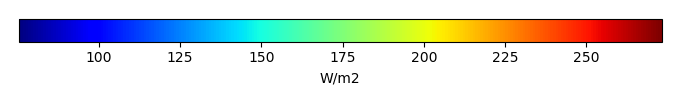MODEL MEANBIAS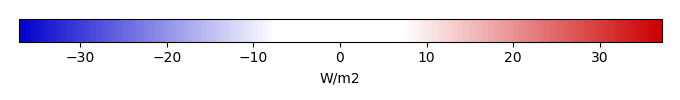BIAS SCORERMSE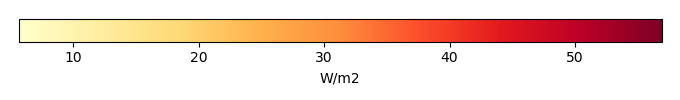RMSE SCOREBENCHMARK INTERANNUAL VARIABILITY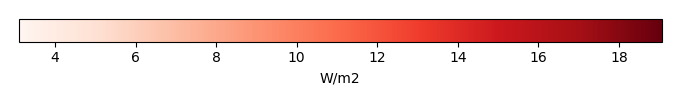MODEL INTERANNUAL VARIABILITYINTERANNUAL VARIABILITY SCOREBENCHMARK MAX MONTHMODEL MAX MONTHDIFFERENCE IN MAX MONTH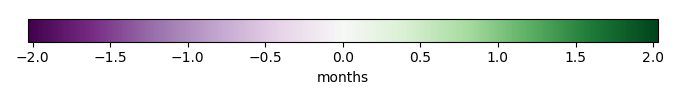SEASONAL CYCLE SCORESPATIAL TAYLOR DIAGRAMMODEL COLORS# Spatially integrated regional mean

MODEL COLORSREGIONAL MEANANNUAL CYCLEMONTHLY ANOMALYANNUAL CYCLE# All Models

BenchmarkCLM5PHSOFFCLM5PHSON# Data Information

creation_date: Thu May 8 23:22:55 PDT 2014

source_file: This product is generated from monthly 1 degree CERES EBAF Radiation observations